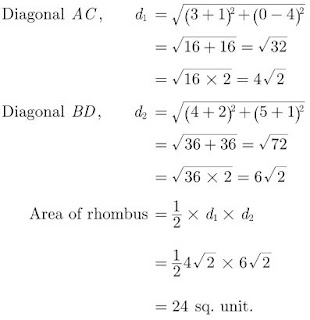>

# Chapter 7 Coordinate Geometry Important Questions for CBSE Class 10 Maths Board Exams

This page contains Important Questions for Class 10 Maths Chapter 7 Coordinate Geometry, which will help the students to prepare for the CBSE Class 10 maths Board exam 2022-23. It help the doing better in their maths paper. Extra questions of Chapter 7 Coordinate Geometry given here which are based on the pattern of CBSE NCERT book. Students will learn about the entire syllabus and learn how to solve problems in preparation for the exams.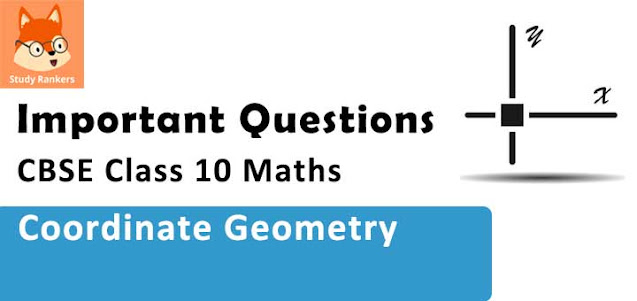## Important Questions for Chapter 7 Coordinate Geometry Class 10 Maths

Important Questions Class 10 Maths contains all types of questions which could be asked in the examination. We have included short answer questions as well as long answer questions for Chapter 7 Coordinate Geometry. For more exercise, students can also refer to the Coordinate Geometry NCERT Solutions. Students can solve these questions and check their answers on the website. All the questions are solved in detail.

### Coordinate Geometry Class 10 Maths Important Questions Very Short Answer (1 Mark)

1. The ordinate of a point A on y-axis is 5 and B has co-ordinates (-3, 1). Find the length of AB.
Solution
We have,
A(0, 5) and B(-3, 1).
Distance between A and B,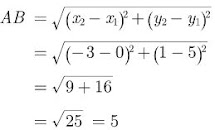2. Write the coordinates of a point P on x-axis which is equidistant from the points A(-2, 0) and B(6,0).

Solution

Since it is equidistant from the points A(-2, 0) and B(6, 0) then

AP = BP

⇒ AP2 = BP2

Using distance formula we have,

[x-(2)]2 + (0-0)2 = (x+6)2 + (0-0)2

⇒ (x+2)2 = (x+6)2

⇒ x2 + 4x + x = x2 + 12x + 36

⇒ 8x = -32

⇒ x = -4

Hence, required point P is (-4, 0).

3. Find the distance of a point P(x,y) from the origin.

Solution

Distance between origin (0, 0) and point P(x,y) is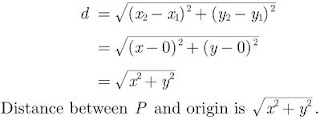4. Find the perpendicular distance o A(5,12) from the y-axis.
Solution
Perpendicular from point (5,12) on y-axis touch it at (0,12).
Distance between (5,12) and (0,12) is,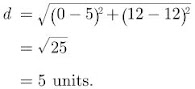5. If the centre and radius of circle is (3, 4) and 7 units respectively, then what it the position of the point A(5, 8) with respect to circle?
Solution
Distance of the point, from the centre,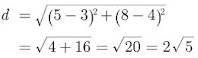Since 2√5 is less than 7, the point lies inside the circle.

6. If the points A(x, 2), B(-3, 4) and C(7, -5) are collinear, then find the value of x.

Solution

When the points are collinear,
x1(y2 – y3) + x2(y3 – y1) + x3(y1 – y2) = 0
⇒ x(-4 – (-5)) + (-3)(-5 – 2) + 7(2 – (-4)) = 0
⇒ x(1) + 21 + 42 = 0
⇒ x + 63 = 0
∴ x = -63

7. For what value of k will k + 9, 2k – 1 and 2k + 7 are the consecutive terms of an A.P.?

Solution

As we know, a2 – a1 = a3 – a2
2k – 1 – (k + 9) = 2k + 7 – (2k – 1)
⇒ 2k – 1 – k – 9 = 2k + 7 – 2k + 1
⇒ k – 10 = 8
∴ k = 8 + 10 = 18

8. ABCD is a rectangle whose three vertices are B(4, 0), C(4, 3) and D(0, 3). Calculate the length of one of its diagonals.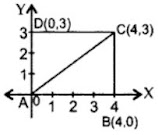Solution

AB = 4 units
BC = 3 units
AC2 = AB2 + BC2 …(Pythagoras’ theorem)
= (4)2 + (3)2
= 16 + 9 = 25
∴ AC = 5 cm

9. If the distance between the points (4, k) and (1, 0) is 5, then what can be the possible values of k.
Solution
Using distance formula we have,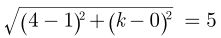32 + k2 = 25
⇒ k2 = 25 - 9 = 16
⇒ k = ±4

### Coordinate Geometry Class 10 Maths Important Questions Short Answer-I (2 Marks)

10. In what ratio does the x -axis divide the line segment joining the points (-4, -6) and (-1, 7)? Find the coordinates of the point of division.
Solution
Let x -axis divides the line-segment joining (-4, -6) and (-1, 7) at the point P(x,y) in the ratio 1:k.
Now, the coordinates of point of division P,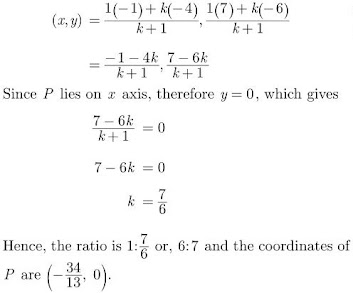11. The x-coordinate of a point P is twice its y-coordinate. If P is equidistant from Q(2, -5) and R(-3, 6), find the co-ordinates of P.

Solution

Let the point P be (2y, y). Since PQ = PR, we have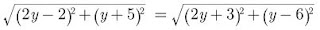Squaring both sides,

(2y-2)2 + (y+5)2 = (2y+3)2 + (y-6)2

⇒ -8y + 4 + 10y + 25 = 12y + 9 -12y + 36

⇒ 2y + 29 = 45

⇒ y = 8

Hence, the coordinates of point P are (16,8).

12. Find the relation between x and y if the points A(x, y), B(-5, 7) and C(-4, 5) are collinear.

Solution

When points are collinear,
∴ Area of ∆ABC = 0
= (x1 (y2 – y3) + x2(y3 – y1) + x3(y1 – y2)) = 0
= x (7 – 5) – 5 (5 – y) -4 (y – 7) = 0
= 2x – 25 + 5y – 4y + 28 = 0
∴ 2x + y + 3 = 0 is the required relation.

13. Find a relation between x and y such that the point P(x, y) is equidistant from the points A (2, 5) and B (-3, 7).

Solution

Let P (x, y) be equidistant from the points A (2, 5) and B (-3, 7).
∴ AP = BP …(Given)
AP2 = BP2 …(Squaring both sides)
(x – 2)2 + (y – 5)2 = (x + 3)2 + (y – 7)2
⇒ x2 – 4x + 4 + y2 – 10y + 25
⇒ x2 + 6x + 9 + y2 – 14y + 49
⇒ -4x – 10y – 6x + 14y = 9 +49 – 4 – 25
⇒ -10x + 4y = 29
∴ 10x + 29 = 4y is the required relation.

14. The points A(4,7), B(p,3) and C (7,3) are the vertices of a right triangle, right-angled at B. Find the value of p.

Solution

As per question, triangle is shown below. Here ABC is a right angle triangle,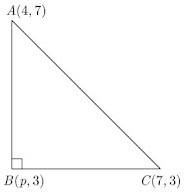AB2 + BC2 = AC2

(p-4)2 + (3-7)2 + (7-p)2 + (3-3)= (7-4)2 + (3-4)2

⇒ (p-4)2 + (-4)2 + (7-p)2 + 0 = 32 + (-4)2

⇒ p2 - 8p + 16 + 16 + 49 + p2 - 14p = 9 + 16

⇒ 2p2 - 22p + 81 = 25

⇒ 2p2 - 22p + 56 = 0

⇒ p2 - 11p + 28 = 0

⇒ (p-4) (p-7) = 0

⇒ p = 7 or 4

15. If A(4,3), B(-1,y) and C(3,4) are the vertices of a right triangle , ABC right angled at A, then find the value of y.

Solution

As per question, triangle is shown below.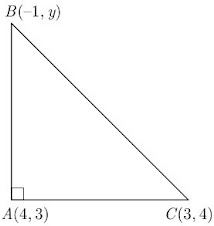Now,

AB2 + AC2 = BC2

(4+1)2 + (3-y)2 + (4-3)2 = (3+1)2 + (4-y)2

⇒ 52 + (3-y)2 + (-1)2 + 12 = 42 + (4-y)2

⇒ 25 + 9 - 6y + y2 + 1 + 1 = 16 + 16 - 8y + y2

⇒ 36 + 2y - 32 = 0

⇒ 2y + 4 = 0

⇒ y = -2

16.  Show that the points (a,a), (-a, -a) and (-√3a, √3a) are the vertices of an equilateral triangle.

Solution

Let A(a,a), B(-a, -a) and C(√3a, √3a)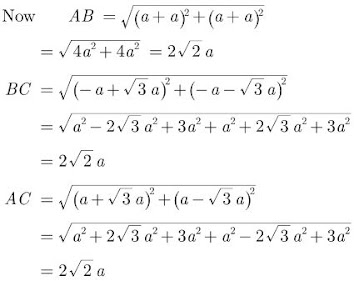Since AB = BC = AC, therefore ABC is an equilateral triangle.

17. If A(4, 3), B(-1, y) and C(3, 4) are the vertices of a right triangle ABC, right-angled at A, then find the value of y.

Solution

We have A(4, 3), B(-1, y) and C(3, 4). In right angled triangle ABC,
(BC)2 = (AB)2 + (AC) …(Pythagoras theorem)
⇒ (-1 – 3)2 + (y – 4)2 = (4 + 1)2 + (3 – y)2 + (4 – 3)2 + (3 – 4)2 …(using distance formula)
⇒ (-4)2 + (y2 – 8y + 16)
⇒ (5)2 + (9 – 6y + y2) + (1)2 + (-1)2
⇒ y2 – 8y + 32 = y2 – 6y + 36 = 0
⇒ -8y + 6y + 32 – 36
⇒ -2y – 4 = 0
⇒ -2y = 4
∴ y = -2

18. If the mid-point of the line segment joining A(x/2, y+1/2) and B(x+1, y-3) is C(5, -2), find x,y.

Solution

If the mid-point of the line segment joining A(x/2, y+1/2) and B(x+1, y-3) is C(5, -2) then at mid point,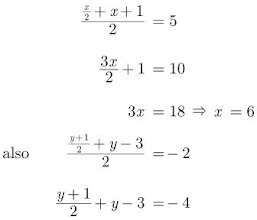⇒ y + 1 + 2y - 6 = -8

⇒ y = -1

19. Show that A(6,4) B(5,-2) and C(7,-2) are the vertices of an isosceles triangle.

Solution

We have A(6,4) B(5,-2) and C(7,-2).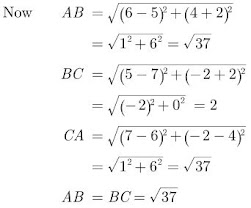Since two sides of a triangle are equal in length, triangle is an isosceles triangle.

20. If the line segment joining the points A(2,1) and B(5,-8) is trisected at the points P and Q, find the coordinates P.

Solution

As per question, line diagram is shown below.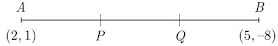Let P(x, y) divides AB in the ratio 1:2.

Using section formula we get,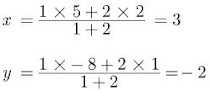Hence, coordinates of P are (3,-2).

21. Three vertices of a parallelogram taken in order are (-1, 0), (3, 1) and (2, 2) respectively. Find the coordinates of fourth vertex. (2011D)

Solution

Let A(-1, 0), B(3, 1), C(2, 2) and D(x, y) be the vertices of a parallelogram ABCD taken in order. Since, the diagonals of a parallelogram bisect each other.
∴ Coordinates of the mid-point of AC = Coordinates of the mid-point of BD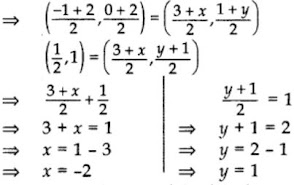Hence, coordinates of the fourth vertex, D(-2, 1).

22. If two adjacent vertices of a parallelogram are (3,2) and (-1,0) and the diagonals intersect at (2,-5) then find the co-ordinates of the other two vertices.

Solution

Let two other co-ordinates be (x,y) and (x',y') respectively using mid-point formula.

As per question parallelogram is shown below.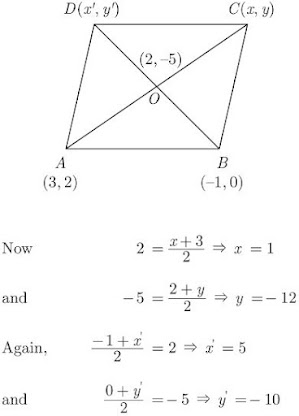Hence, coordinates of C(1,-12) and D(5,-10).

23. If A(5, 2), B(2, -2) and C(-2, t) are the vertices of a right angled triangle with ∠B = 90°, then find the value of t. (2015D)

Solution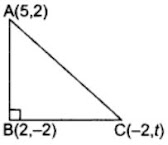ABC is a right angled triangle,
∴ AC2 = BC2 + AB2 …(i) …(Pythagoras theorem)
Using distance formula,
AB2 = (5 – 2)2 + (2 + 2)
= 25
BC2 = (2 + 2)2 + (t + 2)2
= 16 + (t + 2)2
AC2 = (5 + 2)2 + (2 – t)2
= 49 + (2 – t)2
Putting values of AB2, AC2 and BC2 in equation (i), we get
49 + (2 – t)2 = 16 + (t + 2)2 + 25
∴ 49 + (2 – t)2 = 41 + (t + 2)2
⇒ (t + 2)2 – (2 – t)2 = 8
⇒ (t2 + 4 + 4t – 4 – t2 + 4t) = 8
8t = 8
⇒ t = 1

24. If P(2,-1), Q(3,4), R(-2,3) and S(-3,-2) be four points in a plane, show that PQRS is a rhombus but not a square.

Solution

We have,

P(2,-1), Q(3,4), R(-2,3) and S(-3,-2)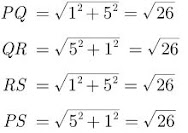Since, all the four sides are equal, PQRS is a rhombus.Since, PQR is not a right triangle, PQRS is a rhombus but not a square.

### Coordinate Geometry Class 10 Maths Important Questions Short Answer-II (3 Marks)

25. Find the ratio in which the segment joining the points (1,-3) and (4,5) is divided by x-axis? Also find the coordinates of this point on x-axis.

Solution

Let the required ratio be k:1 and the point on x-axis be (x,0).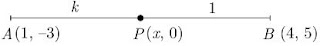Here,

(x1, y1) = (1, -3)

and (x2, y2) = (4,5)

Using section formula y coordinate we obtain,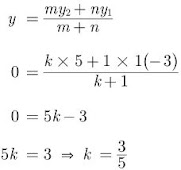Hence, the required ratio is 3/5 i.e. 3:5.

Now, again using section formula for x, we obtain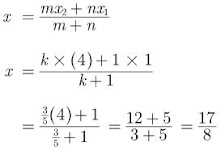Coordinate of P is (117/8, 0).

26. If the point C(-1, 2) divides internally the line segment joining A(2,5) and B(x, y) in the ratio 3 : 4 find the coordinates of B.

Solution

From the given information we have drawn the figure as below.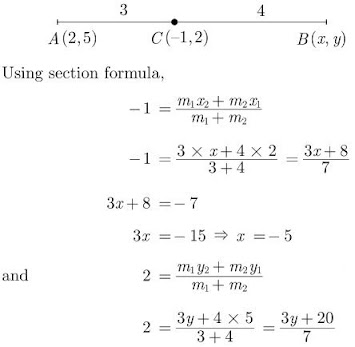3y + 20 = 14

⇒ 3y = 14 - 20 = -6

⇒ y = -2

Hence, the coordinates of B(x, y) is (-5,-2).

27. If the co-ordinates of points A and B are (-2, -2) and (2, -4) respectively, find the co-ordinates of P such that AP = 3/7 AB, where P lies on the line segment AB.

Solution

We have,

AP = 3/7 AB

⇒ AP:PB = 3:4

As per question, line diagram is shown below.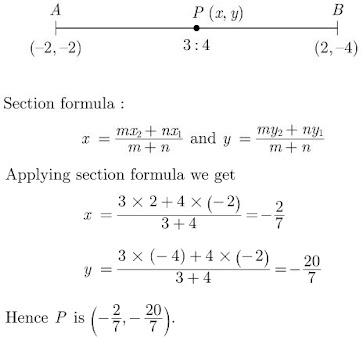28. Find that value(s) of x for which the distance between the points P(x, 4) and Q(9, 10) is 10 units.

Solution

PQ = 10 …(Given)
⇒ PQ2 = 102 = 100 …(Squaring both sides)
(9 – x)2 + (10 – 4)2 = 100 …(using distance formula)
⇒ (9 – x)2 + 36 = 100
⇒ (9 – x)2 = 100 – 36 = 64
⇒ (9 – x) = ± 8 …(Taking square-root on both sides)
⇒ 9 – x = 8 or 9 – x = -8
⇒ 9 – 8 = x or 9+ 8 = x
⇒ x = 1 or x = 17

29. Find the value of y for which the distance between the points A (3,-1) and B (11, y) is 10 units.

Solution

AB = 10 units …(Given)
⇒ AB2 = 102 = 100 …(Squaring both sides)
(11 – 3)2 + (y + 1)2 = 100
⇒ 82 + (y + 1)2 = 100
⇒ (y + 1)2 = 100 – 64 = 36
⇒ y + 1 = ±6 …(Taking square-root on both sides)
⇒ y = -1 ± 6
∴ y = -7 or 5

30. The co-ordinates of the vertices of △ABC are A(7, 2), B(9, 10) and C(1, 4). If E and F are the mid-points of AB and AC respectively, prove that EF = 1/2 BC.

Solution

Let the mid-points of AB and AC be E(x1, y1) and F(x2, y2). As per question, triangle is shown below.31. Find the ratio in which (11, 15) and (9, 20) divides the line segment joining the points (15, 5) and (9, 20).

Solution

Let the two points (15, 5) and (9, 20) are divided in
the ratio : k 1 by point P(11,15).
Using Section formula, we get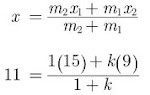⇒ 11 + 11k = 15 + 9k
⇒ k = 2
Thus ratio is 2 : 1.

32. Find the point on y-axis which is equidistant from the points (5, -2) and (-3, 2).

Solution

Let the point be (0,y).

52 + (y+2)2 = 32 + (y-2)2

or, y2 + 25 + 4y + 4 = 9 - 4y + 4

8y = -16 or, y = -2

or, Point (0, -2)

33. The vertices of △ABC are A(6, -2), B(0, -6) and C(4,8). Find the co-ordinates of mid-points of AB, BC and AC.

Solution

Let mid-point of AB, BC and AC be D(x1, y1), E(x2, y2) and F(x3, y3). As per question, triangle is shown below.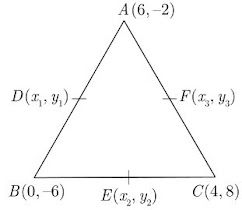Using section formula, the co-ordinates of the points D, E, F are: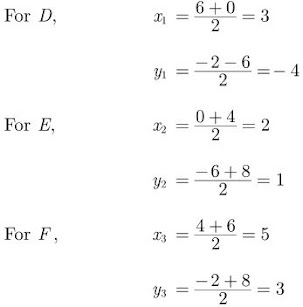The co-ordinates of the mid-points of AB, BC and AC are D(3, -4), E(2,1) and F(5,3) respectively.

34. The point A(3, y) is equidistant from the points P(6, 5) and Q(0, -3). Find the value of y.

Solution

PA = QA …(Given)
⇒ PA2 = QA2 …(Squaring both sides)
(3 – 6)2 + (y – 5)2 = (3 – 0)2 + (y + 3)2
⇒ 9 + (y – 5)2 = 9 + (y + 3)2
⇒ (y – 5)2 = (y + 3)2
⇒ y – 5 = ±(y + 3) …(Taking square root of both sides)
⇒ y – 5 = y + 3 y – 5 = -y – 3
0 = 8 …(not possible)
∴ y = 1

35. If the point P(x, y) is equidistant from the points A(a + b, b – a) and B(a – b, a + b), prove that bx = ay.

Solution

PA = PB …(Given)
PA2 = PB2 …(Squaring both sides)
⇒ [(a+b) – x]2 + [(b-a) – y)2 = [(a–b) – x]2 + [(a+b) – y]2
⇒ (a+b)2 + x2 – 2(a+b)x + (b–a)2 + y2 – 2(b–a)y = (a–b)2 + x2 – 2(a–b)x + (a+b)2 + y2 – 2(a+b)y …[∵ (a–b) 2 = (b–a)2]
⇒ -2(a + b)x + 2(a – b)x = -2(a + b)y + 2(b – a)y
⇒ 2x(-a – b + a – b) = 2y(-a – b + b – a)
⇒ -2bx = –2ay
⇒ bx = ay

36. If the point P(k – 1, 2) is equidistant from the points A(3, k) and B(k, 5), find the values of k.

Solution

PA = PB …(Given)
PA2 = PB2 …(Squaring both sides)
⇒ (k – 1 – 3)2 + (2 – k)2 = (k – 1 – k)2 + (2 – 5)2
⇒ (k – 4)2 + (2 – k)2 = (-1)2 + (-3)2
⇒ k2 – 8k + 16 + 4 + k2 – 4k = 1 + 9
⇒ 2k2 – 12k + 20 – 10 = 0
⇒ 2k2 – 12k + 10 = 0 …(Dividing by 2)
⇒ k2 – 6k + 5 = 0
⇒ k2 – 5k – k + 5 = 0
⇒ k(k – 5) – 1(k – 5) = 0
⇒ (k – 5) (k – 1) = 0
⇒ k – 5 = 0 or k – 1 = 0
∴ k = 5 or k = 1

37. Find the area of the rhombus of vertices (3,0), (4,5), (-1,4) and (-2,-1) taken in order.

Solution

We have,

A(3,0), B(4,5), C(-1,4) and D(-2,-1)